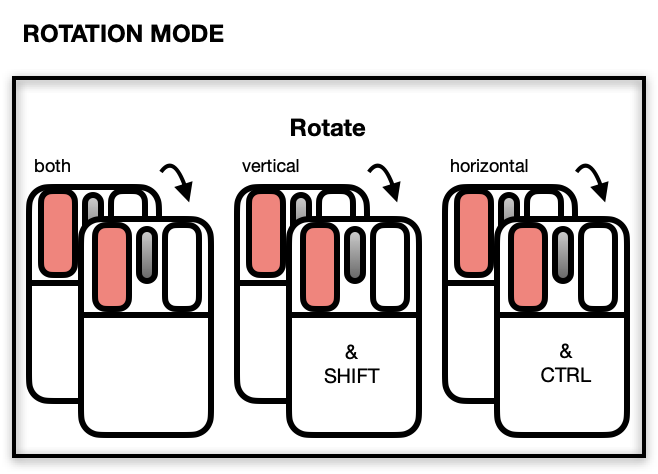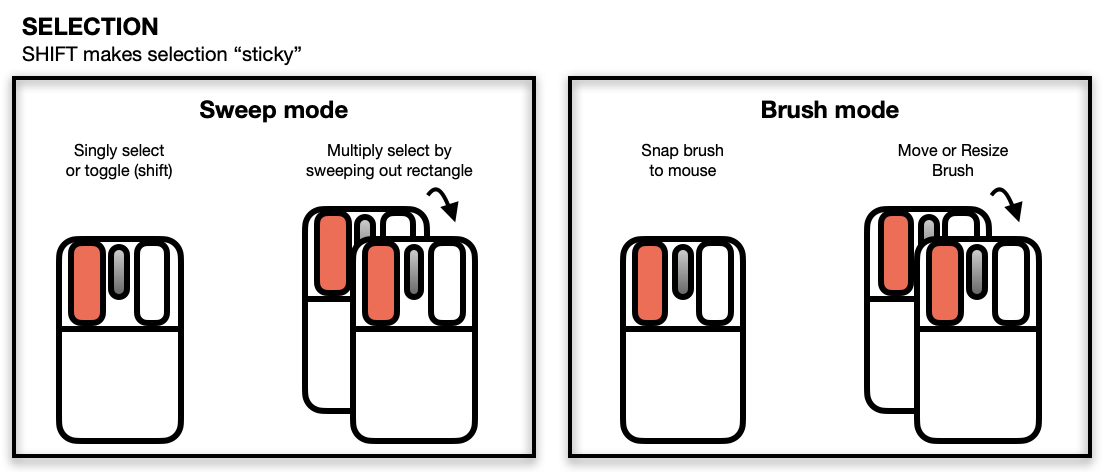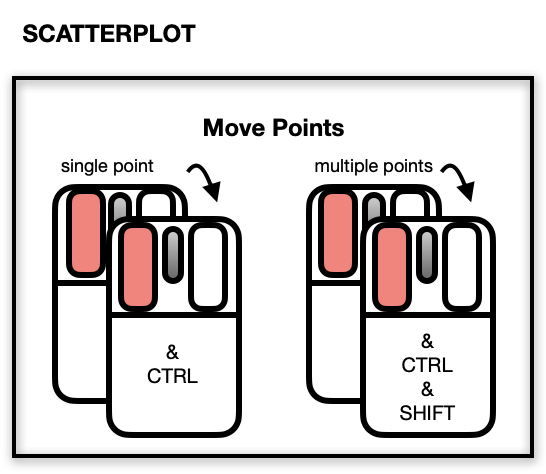l_plot3D is a generic function for creating interactive visualization environments for R objects.

l_plot3D(x, y, z, ...)

# S3 method for default
l_plot3D(
x,
y = NULL,
z = NULL,
axisScaleFactor = 1,
by = NULL,
on,
layout = c("grid", "wrap", "separate"),
connectedScales = c("cross", "row", "column", "both", "x", "y", "none"),
color = l_getOption("color"),
glyph = l_getOption("glyph"),
size = l_getOption("size"),
active = TRUE,
selected = FALSE,
xlabel,
ylabel,
zlabel,
title,
showLabels = TRUE,
showScales = FALSE,
showGuides = TRUE,
guidelines = l_getOption("guidelines"),
guidesBackground = l_getOption("guidesBackground"),
foreground = l_getOption("foreground"),
background = l_getOption("background"),
parent = NULL,
...
)

## Arguments

x the x, y and z arguments provide the x, y and z coordinates for the plot. Any reasonable way of defining the coordinates is acceptable. See the function xyz.coords for details. If supplied separately, they must be of the same length. the y coordinates of points in the plot, optional if x is an appropriate structure. the z coordinates of points in the plot, optional if x is an appropriate structure. named arguments to modify plot states. the amount to scale the axes at the centre of the rotation. Default is 1. All numerical values are acceptable (0 removes the axes, < 0 inverts the direction of all axes.) loon plot can be separated by some variables into multiple panels. This argument can take a formula, n dimensional state names (see l_nDimStateNames) an n-dimensional vector and data.frame or a list of same lengths n as input. if the x or by is a formula, an optional data frame containing the variables in the x or by. If the variables are not found in data, they are taken from environment, typically the environment from which the function is called. layout facets as 'grid', 'wrap' or 'separate' Determines how the scales of the facets are to be connected depending on which layout is used. For each value of layout, the scales are connected as follows: layout = "wrap": Across all facets, when connectedScales is "x", then only the "x" scales are connected "y", then only the "y" scales are connected "both", both "x" and "y" scales are connected "none", neither "x" nor "y" scales are connected. For any other value, only the "y" scale is connected. layout = "grid": Across all facets, when connectedScales is "cross", then only the scales in the same row and the same column are connected "row", then both "x" and "y" scales of facets in the same row are connected "column", then both "x" and "y" scales of facets in the same column are connected "x", then all of the "x" scales are connected (regardless of column) "y", then all of the "y" scales are connected (regardless of row) "both", both "x" and "y" scales are connected in all facets "none", neither "x" nor "y" scales are connected in any facets. colours of points; colours are repeated until matching the number points. Default is found using l_getOption("color"). the visual representation of the point. Argument values can be any of the string names of primitive glyphs: circles: "circle", "ccircle", "ocircle"; squares or boxes: "square", "csquare", "osquare"; triangles: "triangle", "ctriangle", "otriangle"; diamonds: "diamond", "cdiamond", or "odiamond". Note that prefixes "c" and "o" may be thought of as closed and open, respectively. The set of values are returned by l_primitiveGlyphs(). the string names of constructed glyphs: text as glyphs: see l_glyph_add_text() point ranges: see l_glyph_add_pointrange() polygons: see l_glyph_add_polygon() parallel coordinates: see l_glyph_add_serialaxes() star or radial axes: see l_glyph_add_serialaxes() or any plot created using R: see l_make_glyphs() Note that glyphs are constructed and given a stringname to be used in the inspector. size of the symbol (roughly in terms of area). Default is found using l_getOption("size"). a logical determining whether points appear or not (default is TRUE for all points). If a logical vector is given of length equal to the number of points, then it identifies which points appear (TRUE) and which do not (FALSE). a logical determining whether points appear selected at first (default is FALSE for all points). If a logical vector is given of length equal to the number of points, then it identifies which points are (TRUE) and which are not (FALSE). Label for the horizontal (x) axis. If missing, one will be inferred from x if possible. Label for the vertical (y) axis. If missing, one will be inferred from y (or x) if possible. Label for the third (perpendicular to the screen) (z) axis. If missing, one will be inferred from z (or x) if possible. Title for the plot, default is an empty string. logical to determine whether axes label (and title) should be presented. logical to determine whether numerical scales should be presented on both axes. logical to determine whether to present background guidelines to help determine locations. colour of the guidelines shown when showGuides = TRUE. Default is found using l_getOption("guidelines"). colour of the background to the guidelines shown when showGuides = TRUE. Default is found using l_getOption("guidesBackground"). foreground colour used by all other drawing. Default is found using l_getOption("foreground"). background colour used for the plot. Default is found using l_getOption("background"). a valid Tk parent widget path. When the parent widget is specified (i.e. not NULL) then the plot widget needs to be placed using some geometry manager like tkpack or tkplace in order to be displayed. See the examples below.

## Value

if the argument by is not set, a loon widget will be returned; else an l_facet object (a list) will be returned and each element is a loon widget displaying a subset of interest.

## Details

To get started with loon it is recommended to read loons website which can be accessed via the l_help() function call.

The general direct manipulation and interaction gestures are outlined in the following figures.

Rotating

Press 'R' to toggle rotation mode. When rotation mode is active, either use the below mouse gestures or arrow keys to rotate the plot.The centre of the rotation can be changed by panning the plot. To reset the rotation, use the tripod icon in the plot inspector.

Zooming and PanningSelecting Points/ObjectsMoving Points on the Scatterplot DisplayNOTE: Although it is possible to programmatically add layers to an l_plot3D, these will not appear as part of the 3D plot's display. There is no provision at present to incorporate rotation of 3D geometric objects other than point glyphs.

The scatterplot displays a number of direct interactions with the mouse and keyboard, these include: rotating, zooming towards the mouse cursor using the mouse wheel, panning by right-click dragging and various selection methods using the left mouse button such as sweeping, brushing and individual point selection. See the documentation for l_plot3D for more details about the interaction gestures.

Turn interactive loon plot static loonGrob, grid.loon, plot.loon.

Other three-dimensional plotting functions: l_scale3D()

## Examples

if(interactive()){

with(quakes,
l_plot3D(long, lat, depth, linkingGroup = "quakes")
)

with(l_scale3D(quakes),
l_plot3D(long, lat, depth, linkingGroup = "quakes")
)

scaled_quakes <- l_scale3D(quakes)
with(scaled_quakes,
l_plot3D(long, lat, depth, linkingGroup = "quakes")
)

with(scaled_quakes,
l_plot3D(mag, stations, depth, linkingGroup = "quakes")
)

# Or together:
with(scaled_quakes,{
l_plot3D(long, lat, depth, linkingGroup = "quakes")
l_plot3D(mag, stations, depth, linkingGroup = "quakes")
}
)

}
if(interactive()){

# default use as scatterplot

p1 <- with(quakes,
l_plot3D(long, lat, depth)
)

p2 <- with(quakes,
l_plot3D(mag, stations, depth)
)

# link the two plots p1 and p2
l_configure(p1, linkingGroup = "quakes", sync = "push")
l_configure(p2, linkingGroup = "quakes", sync = "push")

}